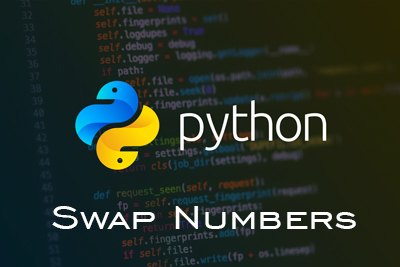Swap Numbers - Python CBSE
12-09-2020    87 times### Write a program in Python to swap two given numbers.

Swap numbers is very easy in python.

```	i=int(input("Enter First number :"))
j=int(input("Enter Second number :"))
print("i=",i," and j=",j)
i,j=j,i
print("i=",i," and j=",j)
```